## Thursday, 17 October 2013

### Techniques to improve the accuracy of your Predictive Models

I did a presentation at the MelbURN R User Group last night on a few techniques to improve the accuracy of your predictive models and also talked a bit about the Heritage Health Prize story and how our win unfolded. The video of the talk is below and the R code I used in the simulations below that.

You will probably be able to enhance the code and make it more efficient. If you do, please let us all know.

Thanks for all your positive feedback - hope you all had an enjoyable night.

# Code from the presentation

## Footy Tipping

``````#--------------------------------------
# Footy Tipping Simulation
# Ensembling of Tipsters
#
# Phil Brierley
# Oct 2013
#---------------------------------------

#clean all objects from memory
rm(list=ls())

#------------------------------
# adjustable parameters to set
#------------------------------
Number_of_Tipsters <- 12
Number_of_Games <- 2000
Number_ofSeasons <- 100
Tipster_Strength <- 0.6
#------------------------------

#simulation each season
for (Season in 1:Number_ofSeasons){

#gernerate random tips
Results = matrix(rbinom(Number_of_Games*Number_of_Tipsters,1,Tipster_Strength),Number_of_Games,Number_of_Tipsters)

#majority vote = median score
Results[,Number_of_Tipsters] <- apply(Results[,1:(Number_of_Tipsters-1)],1,median)

#find the mean score per tipster over the season
seasonSummary <- apply(Results,2,mean)

#stack the seasons together
if (Season == 1) {
runningSummary <- seasonSummary
} else {
runningSummary <- rbind(runningSummary,seasonSummary)
}

} #Number_ofSeasons

#give the columns sensible names
colnames(Results)[1:Number_of_Tipsters] <- paste('Tipster',1:Number_of_Tipsters)
colnames(Results)[Number_of_Tipsters] <- paste('Majority Vote')
colnames(runningSummary) <- colnames(Results)

#plot the results
bestPunter <-  apply(runningSummary[,1:(Number_of_Tipsters-1)],1,max)

plot(runningSummary[,Number_of_Tipsters]
,type='l'
,col='red'
,ylim=c(Tipster_Strength - 0.1,1)
,xlab='Season'
,ylab='% of Games Correct')

lines(bestPunter,col='blue')
abline(h=Tipster_Strength,col='green')
bp <- mean(bestPunter)
abline(h=bp,col='blue')
mv <- mean(runningSummary[,Number_of_Tipsters])
abline(h=mv,col='red')

legend("topright"
, inset=.05
, c(paste("Majority Vote (avg=",mv,")"),paste("Best Tipster (avg=",bp,")"),paste('expected (',Tipster_Strength,')'))
,fill=c('red','blue','green')
,horiz=FALSE)
``````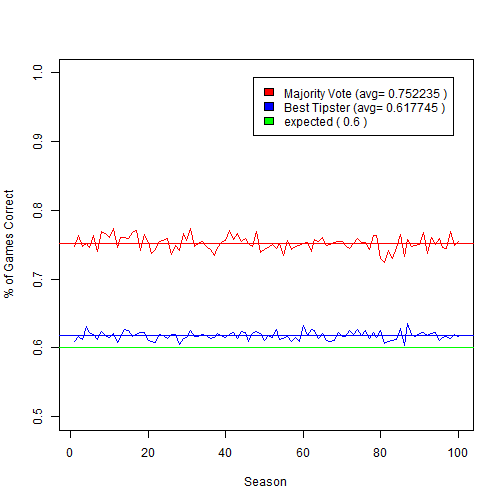## Footy Tipping Parallel

``````I think there is a 'parallel' package in base R now also.
library(help = "parallel")
``````
``````
###############################
# Footy Tipping Simulation
# Ensembling of Tipsters
#
# Parallel Version
#
# Phil Brierley
# Oct 2013
#
###############################

#clean all objects from memory
rm(list=ls())

#set memory
memsize <- 3200
if (memory.limit() < memsize)  memory.limit(size=memsize)

#----------------------
#parameters to set
#-----------------------
Number_of_Tipsters <- 12
Number_of_Games <- 20000
Number_ofSeasons <- 100
Tipster_Strength <- 0.6
threads <- 8 #depends on how many processors you have

#----------------------------------------------
# main function to simulate a season
#----------------------------------------------
simulateSeason <- function(f){

#gernerate random tips
Results = matrix(rbinom(Number_of_Games*Number_of_Tipsters,1,Tipster_Strength),Number_of_Games,Number_of_Tipsters)

#my tip is a majority vote - hence median score
Results[,Number_of_Tipsters] <- apply(Results[,1:(Number_of_Tipsters-1)],1,median)

#find the mean score per tipster over the season
seasonSummary <- apply(Results,2,mean)

}
#end of function
#---------------------------------------------

#--------------------------------
# the parallel stuff
#--------------------------------

library(snowfall)

#initiate clusters
sfStop()
sfInit(parallel = TRUE, cpus = threads, type = "SOCK")
sfExport(list = c("Number_of_Games","Number_of_Tipsters","Tipster_Strength"))

#start the clock
timeStart <- Sys.time()

#do the calculation in parallel
seasonSummary <- sfClusterApplyLB(1:Number_ofSeasons, simulateSeason)

#stack results together into a data frame
runningSummary <- do.call(rbind.data.frame, seasonSummary)
colnames(runningSummary) <- paste('punter',1:ncol(runningSummary))
colnames(runningSummary)[ncol(runningSummary)] <- paste('ensemble')

#record the time it took
totTime <-  as.numeric(Sys.time() - timeStart, units = "secs")
myText <- paste('Avg calculation time per season = ', formatC(totTime/ Number_ofSeasons,digits=2,format='f') ,'seconds')

#stop clusters
sfStop()

#--------------------------------------
#plot the results
#--------------------------------------
bestPunter <-  apply(runningSummary[,1:(Number_of_Tipsters-1)],1,max)
plot(runningSummary[,Number_of_Tipsters]
,type='l'
,col='red'
,ylim=c(Tipster_Strength - 0.1,1)
,xlab='Season'
,ylab='% of Games Correct'
,main=myText)

lines(bestPunter,col='blue')
abline(h=Tipster_Strength,col='green')
bp <- mean(bestPunter)
abline(h=bp,col='blue')
mv <- mean(runningSummary[,Number_of_Tipsters])
abline(h=mv,col='red')

legend("topright"
,inset=.05
,c(paste("Majority Vote (avg=",mv,")")
,paste("Best Tipster (avg=",bp,")")
,paste('expected (',Tipster_Strength,')'))
,fill=c('red','blue','green')
,horiz=FALSE)
``````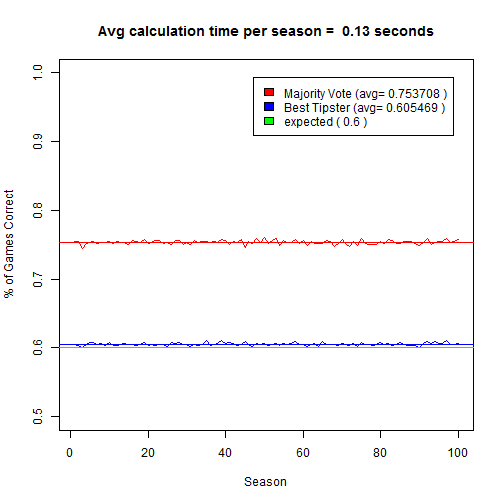## Model Synergy

``````
###############################################
# If two models have the same RMSE error
# but are different, what do we get by
# averaging them?
#
# Phil Brierley
# Oct 2013
#
###############################################

#clean all objects from memory
rm(list=ls())

#number of cases
cases <- 100000

#how much worse is one model than the other
worseness_factor = 1.0

#generate random errors
errors1 <- rnorm(cases,0,1)
errors2 <- rnorm(cases,0,1) * worseness_factor

#average the 2
errorsAve <- (errors1 + errors2)/2

#calculate then RMSE
rmse1 <-  sqrt(sum(errors1 * errors1)/cases)
rmse2 <-  sqrt(sum(errors2 * errors2)/cases)
rmseAve <-  sqrt(sum(errorsAve * errorsAve)/cases)

#-----------------------------
# plot the results
#-----------------------------

op <- par(mfrow=c(2,2))

#histogram of errors
bp <- barplot(c(rmse1,rmse2,rmseAve)
,ylim =c(0,1.1*max(rmse1,rmse2,rmseAve))
,ylab='rmse')
axis(side = 1, at = bp, labels = c('model1','model2','modelAverage'))
abline(h=rmseAve,col='red',lwd=4)

#lineplot of errors
num <- 25
plot(errors1[1:num]
,col='red'
,type='l'
,ylim=c(min(errors1,errors2),max(errors1,errors2))
,ylab='error'
,xlab='case')
lines(errors2[1:num],col='blue',type='l')
lines(errorsAve[1:num],col='forestgreen',type='l',lwd=3)
abline(h=0)

#error distribution
br <- seq(from=min(errorsAve,errors2),to=max(errorsAve,errors2),length.out=100)
hist(errorsAve,breaks=br,col=rgb(0,0,1,1/4),xlab='error',main='error distribution')
legend("topright", inset=.05, c('model1','averaged models'), fill=c(rgb(1,0,0,1/4),rgb(0,0,1,1/4)), horiz=FALSE)

#scatterplot of errors
plot(errors1,errors2,main=paste('correlation=',cor(errors1,errors2)),xlab='model1 errors',ylab='model2 errors')
``````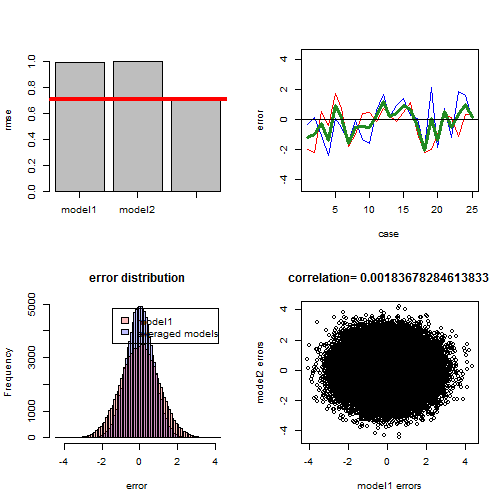``````par(op)
``````

## Model Synergy but varying correlation

``````###############################################
# If two models have the same RMSE error
# but are different, what do we get by
# averaging them?
#
# What happens as the correlation between the
# two models changes
#
# Phil Brierley
# Oct 2013
#
###############################################

#clean all objects from memory
rm(list=ls())
#memsize <- 6400
#if (memory.limit() < memsize)  memory.limit(size=memsize)

#settings
cases <- 1000
number_of_correlations_to_test <- 20
worseness_factor = 1.3

#initiate
errors1 <- rnorm(cases,0,1)
correlations <- seq(from=0,to=1, length.out = number_of_correlations_to_test)

#-----------------------------------------------------------------
# function to generate a correlated variable
#-----------------------------------------------------------------
generateCorrelatedVariable <- function(x1,rho){

if (rho==1) return(x1)

n     <- length(x1)                    # length of vector
#rho   <- 0.8                   # desired correlation = cos(angle)
theta <- acos(rho)             # corresponding angle
#x1    <- rnorm(n, 1, 1)        # fixed given data
x2    <- rnorm(n, 1, 1)      # new random data
X     <- cbind(x1, x2)         # matrix
Xctr  <- scale(X, center=TRUE, scale=FALSE)   # centered columns (mean 0)

Id   <- diag(n)                               # identity matrix
Q    <- qr.Q(qr(Xctr[ , 1, drop=FALSE]))      # QR-decomposition, just matrix Q
P    <- tcrossprod(Q)          # = Q Q'       # projection onto space defined by x1
x2o  <- (Id-P) %*% Xctr[ , 2]                 # x2ctr made orthogonal to x1ctr
Xc2  <- cbind(Xctr[ , 1], x2o)                # bind to matrix
Y    <- Xc2 %*% diag(1/sqrt(colSums(Xc2^2)))  # scale columns to length 1

x <- Y[ , 2] + (1 / tan(theta)) * Y[ , 1]     # final new vector

}

#---------------------------------------------------------------------
# function to calculate the errors
#---------------------------------------------------------------------
calcErrors <- function(myCorrelation){

errors2 <- worseness_factor * scale(generateCorrelatedVariable(errors1,myCorrelation))

#average the 2
errorsAve <- (errors1 + errors2)/2

#calculate then RMSE
cases <- length(errors1)
rmse1 <-  sqrt(sum(errors1 * errors1)/cases)
rmse2 <-  sqrt(sum(errors2 * errors2)/cases)
rmseAve <-  sqrt(sum(errorsAve * errorsAve)/cases)

#return the results
c(myCorrelation,rmse1,rmse2,rmseAve)
}

#--------------------------------
# the parallel stuff
#--------------------------------

library(snowfall)

#initiate clusters
sfStop()
sfInit(parallel = TRUE, cpus = threads, type = "SOCK")

sfExport(list = c("errors1","generateCorrelatedVariable","calcErrors","worseness_factor"))

#do the calculation in parallel
allErrors <- sfClusterApplyLB(correlations,calcErrors)

#stack results together into a data frame
allErrors <- do.call(rbind.data.frame, allErrors)

colnames(allErrors) <- c('correlation','error1','error2','errorAve')

#stop clusters
sfStop()

#------------------------------------------
# plot the results
#------------------------------------------
yrange <- c(min(allErrors\$errorWeighted,allErrors\$errorAve,allErrors\$error1,allErrors\$error2),max(allErrors\$errorWeighted,allErrors\$errorAve,allErrors\$error1,allErrors\$error2))
plot(allErrors\$correlation,allErrors\$errorAve
,type='b'
,col='red'
,lwd=3
,xlab='correlation between the two models'
,ylab='RMS Error'
,main='The less correlated the two models, the more synergy when averaged'
,ylim=yrange)
lines(allErrors\$correlation,allErrors\$error1,col='blue',type='b')
lines(allErrors\$correlation,allErrors\$error2,col='black',type='b')
legend("left", inset=.05, c('model1','model2','average'), fill=c('blue','black','red'), horiz=FALSE)
``````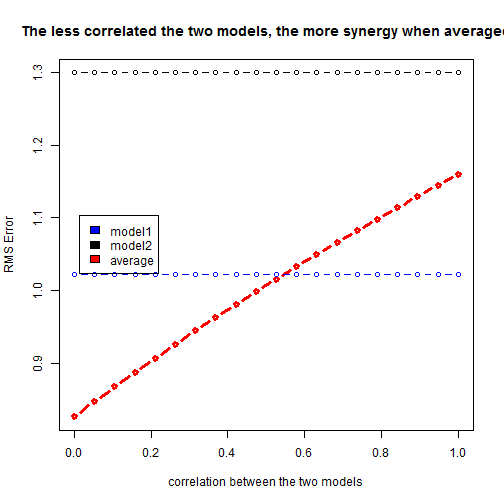## Variable Importance

``````#####################################################
#
# A generic method to calculate the importance
# of variables in any model
#
# Phil Brierley
# Oct 2013
#
#####################################################

#clean all objects from memory
rm(list=ls())

#set memory
memsize <- 3200
if (memory.limit() < memsize)  memory.limit(size=memsize)

#libraries
library(nnet)
library(randomForest)
library(gbm)

#----------------------
#parameters to set
#-----------------------

#what model are we building
modTypes <- vector()
modTypes = 'linear_regression'
modTypes = 'neural_net'
modTypes = 'gbm'
modTypes = 'random_forest'

#a number >-=0 and < 1
deletion_threshold <- 0.05

#for data set generation
Number_of_Useful_Variables <- 10
Number_of_Junk_Variables <- 10
Number_of_Records <- 1000
Number_of_Removed_Useful_Variables <- 0
Include_Junk_Variables <- TRUE

#importance testing loops
numloopsImportance <- 100

#train test split
Train_Percent <- 0.5

#-----------------------------------------
# error function
#-----------------------------------------
calc_error <- function(act,pred){

aact <- as.matrix(act)
ppred <- as.matrix(pred)

return (sqrt(colSums(((ppred) - (aact)) ^ 2) / nrow(aact)))

}

#------------------------
#generate a data set
#------------------------

#set seed if you want to regenerate the same data set
set.seed(42)

useful <- matrix(runif(Number_of_Records*Number_of_Useful_Variables,0,1),Number_of_Records,Number_of_Useful_Variables)
junk <- matrix(runif(Number_of_Records*Number_of_Junk_Variables,0,1),Number_of_Records,Number_of_Junk_Variables)

colnames(useful) <- paste('useful',1:ncol(useful),sep="_")
colnames(junk) <- paste('junk',1:ncol(junk),sep="_")

#create the target
useful_weightings <- sort(runif(Number_of_Useful_Variables,0,1),decreasing=TRUE)
target <- useful %*% useful_weightings

#remove some useful variables
useful <- useful[,1:(Number_of_Useful_Variables-Number_of_Removed_Useful_Variables)]

#create a data set
if (Include_Junk_Variables){
myData <- data.frame(cbind(useful,junk,target))
} else {
myData <- data.frame(cbind(useful,target))
}

#target - what we are predicting
theTarget <- 'target'

targindex <- ncol(myData)
colnames(myData)[targindex] <- theTarget

#----------------------------------------------------
# divide data set into train and test
#----------------------------------------------------
trainrows <- runif(nrow(myData)) < Train_Percent
if(length(which(trainrows)) < 2) stop('not enough training cases')
testrows <- !trainrows

#-------------------------------------------------
# function for calculating variable importance
#--------------------------------------------------

varImporatnce <- function(variable){

#initialse the errors
errorTrain <- 0
errorTest <- 0

#copy this variable data
temp <- myData[,variable]

for(i in 1:numloopsImportance){

#scramble the values of this variable
myData[,variable] <- temp[order(runif(length(temp)))]

#calculate the predictions
if (modType == 'neural_net'){
predictions <- predict(model,newdata=myData[,-targindex],type='raw')
}

if (modType == 'linear_regression'){
predictions <- predict(model, myData)
}

if (modType == 'random_forest'){
predictions <- predict(model, myData,type="response")
}

if (modType == 'gbm'){
predictions <- predict.gbm(model, myData[,-targindex],type="response",n.trees = model\$n.trees)
}

#calculate the error
errorTest <- errorTest + calc_error(myData[testrows,theTarget],predictions[testrows])
errorTrain <- errorTrain + calc_error(myData[trainrows,theTarget],predictions[trainrows])
}

#return average train and test error
c(errorTrain/numloopsImportance,errorTest/numloopsImportance)

}

#----------------------------------------
# set up multithreading
#---------------------------------------
library(snowfall) #for parallel processing
library(rlecuyer)
sfInit(parallel = TRUE, cpus = threads, type = "SOCK")
sfClusterSetupRNG()

sfExport(list = c('myData','trainrows','testrows','numloopsImportance','calc_error','theTarget','targindex'))

####################################
# LOOP THROUGH ALL MODEL TYPES
####################################

variables <- setdiff(colnames(myData),theTarget)
candidates_for_deletion <- NULL

for (modType in modTypes){

#-----------------------------
#build a model
#----------------------------

if (modType == 'linear_regression'){
model <- lm(as.formula(paste(theTarget, " ~ . "))
, data=myData[trainrows,])
basePredictions <-  predict(model, myData)
}

if (modType == 'neural_net'){
model <- nnet(x=myData[trainrows,-targindex]
,y=myData[trainrows,targindex]
,size=5
,linout=TRUE)
basePredictions <- predict(model,newdata=myData[,-targindex],type='raw')

}

if (modType == 'random_forest'){
model <- randomForest(x= myData[trainrows,-targindex]
,y=myData[trainrows,targindex]
,ntree=1000)
basePredictions <-  predict(model,myData,type="response")
}

if (modType == 'gbm'){
model <- gbm(as.formula(paste(theTarget, " ~ . ")),         # formula
data=myData[trainrows,],                   # dataset
distribution="gaussian",     # see the help for other choices
n.trees=1000,                # number of trees
shrinkage=0.05,              # shrinkage or learning rate,
keep.data=FALSE,              # keep a copy of the dataset with the object
verbose=FALSE,               # don't print out progress
n.cores=1)                   # use only a single core (detecting #cores is # error-prone, so avoided here)

basePredictions <- predict.gbm(object=model, newdata=myData[,-targindex],type="response",n.trees = model\$n.trees)

}

#calculate the error
full_Train_Error <- calc_error(myData[trainrows,theTarget],basePredictions[trainrows])
full_Test_Error <- calc_error(myData[testrows,theTarget],basePredictions[testrows])

#Export model to threads
sfExport(list = c('modType','model'))
if (modType == 'neural_net') sfLibrary(nnet)
if (modType == 'random_forest') sfLibrary(randomForest)
if (modType == 'gbm') sfLibrary(gbm)

#-------------------------------------
# calculate variable importance
#-------------------------------------
s <- sfClusterApplyLB(variables,varImporatnce)
s <- do.call(rbind.data.frame,s)
colnames(s) <- c('Train','Test')
row.names(s) <- variables

#get the full model error
s\$Train <- s\$Train / full_Train_Error
s\$Test <- s\$Test / full_Test_Error

#scale to 0-1
myRows <- which(s\$Train > 1)
s[myRows,c('Train')] <- s[myRows,c('Train')] / max(s\$Train)
s[-myRows,c('Train')] <-0

myRows <- which(s\$Test > 1)
s[myRows,c('Test')] <- s[myRows,c('Test')] / max(s\$Test)
s[-myRows,c('Test')] <-0

#pick candidates for deletion based on a threshold
my_candidates_for_deletion <- rownames(s[which(s\$Test < deletion_threshold),])
candidates_for_deletion <- c(my_candidates_for_deletion,candidates_for_deletion)

#get the ranking of each variable
s1 <- s
s1 <- s1[order(s1\$Test,decreasing = TRUE),]
s1\$Rank <- 1:nrow(s1)
colnames(s1)[ncol(s1)] <- paste('Rank',modType,sep="_")
s1 <-s1[order(row.names(s1)),]

#combine the rankings
if (modType == modTypes){
rankings <- s1[ncol(s1)]
} else {
rankings <- cbind(rankings,s1[ncol(s1)])
}

#---------------------------------------
# plot the chart
#---------------------------------------
s <- s[order(s\$Test),]
x <- barplot(as.matrix(t(s))
,horiz=TRUE
,beside=TRUE
,main = paste(modType,'variable importance\nTrain RMSE =', formatC(full_Train_Error,digits = 5,format='f'),'\nTest RMSE = ',formatC(full_Test_Error,digits = 5,format='f'))
,col=c("aliceblue","forestgreen")
,xlim=c(-0.2,1)
,axes = FALSE
,axisnames = FALSE)
text(-0.1,colSums(x)/2,row.names(s),col='blue')
legend('bottomright',inset=0.05,c('Train','Test'),fill=c("aliceblue","forestgreen"))
abline(v=0)

} #end looping through model types
``````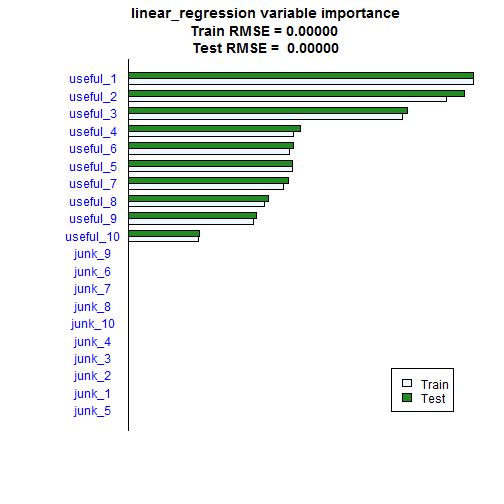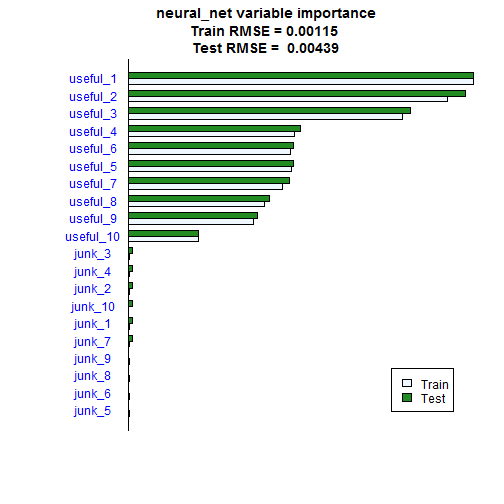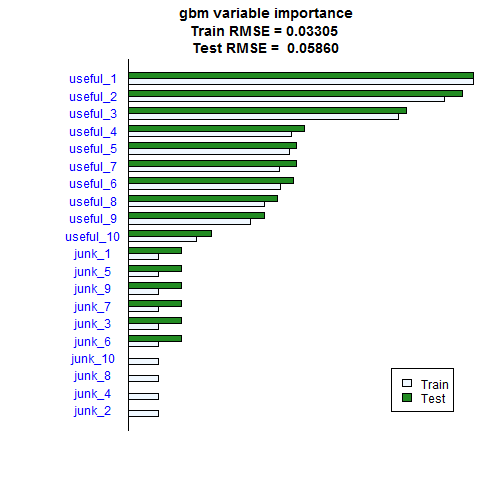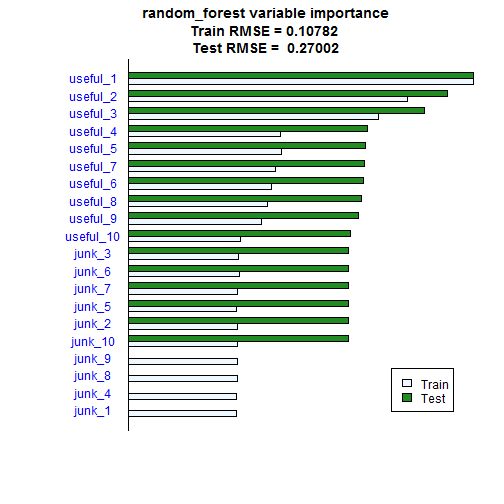``````

sfStop()

#plot the candidates for deletion
``````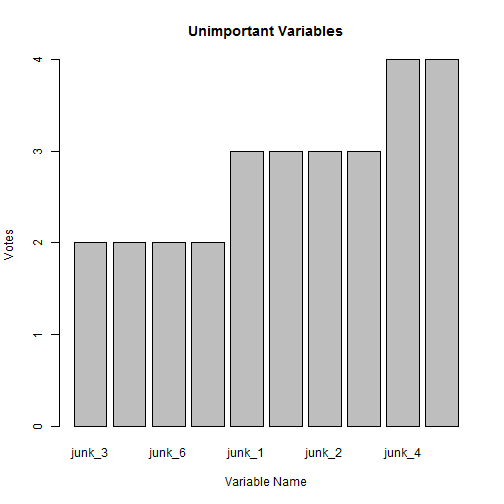``````
#plot average ranking
d <- sort(rowMeans(rankings),decreasing = TRUE)
x <- barplot(
d
,main='Average Variable Ranking'
,ylab='Variable'
,xlab='Average Rank'
,horiz=TRUE
,xlim=c(-3,max(d))
,axes = TRUE
,axisnames = FALSE
)
text(-1.5,x,names(d),col='blue')
text(0.5,x+0.05,formatC(d,digits = 1,format='f'),col='blue',cex=0.8)
``````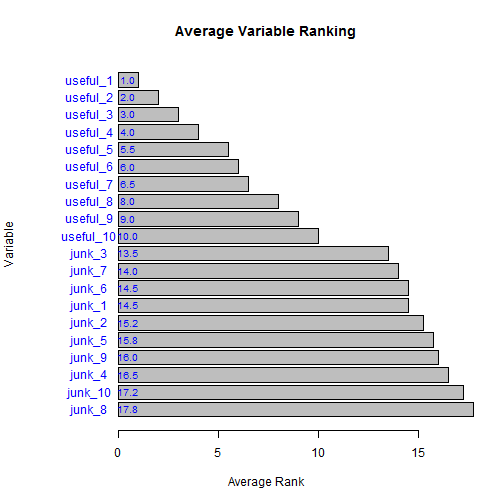1.Excellent talk !

Curious of something in relation to the variable importance bit: It seemed you were advocating using all of your learners to vote on important variables. If so, then do you use this important set to refit all the models using only this predictor set? Doesn't that impact the ability of the disparate models when ensembling together?

By the way, http://cran.r-project.org/web/packages/RRF/index.html appears to be a published R package for regularized random forest. Also: https://dl.dropboxusercontent.com/u/45301435/GRF.pdf

Jeff

2.Hi Jeff,
I wasn't particularly advocating anything in particular - just wanted to demonstrate how this technique was algorithm independent and plot a few charts - the voting stuff was just one of those charts.
In reality what you could do is stick in a random number as a variable and use this as the cut off point. Any variables that are no more important than the random number across all algorithms can probably be eliminated - although this was hard to do in this demo as the numbers were random anyway.
The regularised random forest is not the same algorithm as Rie's, although thanks for introducing it to me.

1.Thanks for the clarification.

2.Hi Phil
Great talk, I have shared this with tthe modellers in my team. I'm curious why you perturb the data rather than leave each variable out? Most documented approaches I've seen so the later. Is that something you've seen elsewhere and what are the advantages?

3.Hi Shane,
This method gives an indication of the variable importance in the model we've built - so if it is your final model then it indicates the variables driving that model. In order to leave a variable out you need to build a model for each variable. My method saves you having to do this and will quickly indicate all the variables that can be eliminated as they are having no effect on the outcome of that model.

The variable importance will change depending on the variables in the model. For example, if two variables are essentially the same then they could share the importance wheras if we got rid of one of them it could suddenly become twice as important. If

This technique is not new. see Elements of Statistical Learning, bottom of page 593.
http://www-stat.stanford.edu/~tibs/ElemStatLearn/

3.Got it, thanks!

4.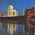This comment has been removed by a blog administrator.

5.I enjoyed your presentation thank you. There were some good tips. Another tip would be to create new features using existing features. eg. feature9 = feature1 * feature5.

Kind Regards,

James

6.I enjoyed it its i like it.
Coal Mines

7.This was a really informative article

8.This comment has been removed by the author.

9.This comment has been removed by a blog administrator.

10.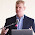This comment has been removed by a blog administrator.

11.Thanks For this information. Data Mining is known as the process of extracting the useful information from a pool of data and transforming them into a required format like CSV, Excel, HTML etc.

12.Good post.

13.Very nice article. Was there ever parts 2 & 3. I'd be interested in see those.

14.sorry, no. But I am putting together some tutorials if you are Melbourne based.

15.Really good article/speech. I love the method of evaluating feature importance. Does this method have a name or any other reference?

(BTW: It was a bit unclear to me at first weather or not you retrained the model in between each feature shuffling. Apparently you do not retrain, but only consider the a pretrained model. If you had retrained the model it would be similar to one step of RFE.)

1.Well... If I'd just read the other comments, I would have seen that you are referring to Elements of Statistical Learning 2ed. bottom of page 953. An updated link here: https://web.stanford.edu/~hastie/Papers/ESLII.pdf

16.This blog is having advanced information about the techniques of the Data Mining . Very nice and thank you for sharing such information.

17.COEPD LLC- Center of Excellence for Professional Development is the most trusted online training platform to global participants. We are primarily a community of Business Analysts who have taken the initiative to facilitate professionals of IT or Non IT background with the finest quality training. Our trainings are delivered through interactive mode with illustrative scenarios, activities and case studies to help learners start a successful career. We impart knowledge keeping in view of the challenging situations individuals will face in the real time, so that they can handle their job deliverables with at most confidence.

18.Data Mining Services

The precise understanding of your collected data can actually lead to quality data processing, better decision making, customer satisfaction and obviously improved company’s revenue. This is where the role of Data Mining fits in to improve a business’ efficiency with a positive approach. It is a process that aims at identifying new, meaningful and purposeful data in your existing accumulated data. This data is further utilized to deal with competitive business industry with a foresight and valuable interference.

Along with the professional and dedicated team of Data Miners, we at Loginworks hold the expertise in converting a huge amount of raw data into a valuable information. This process may appear time-taking, but it eventually helps in making strategic business decisions with an ease. With an experience of 11 years of experience in our domain, we ensure that the data reaches in an accurate and fast manner to our clients.
data mining services

19.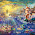Thanks for sharing the descriptive information on MSBI Tutorial.It’s really helpful to me since I'm taking MSBI Training. Keep doing the good work and if you are interested to know more on MSBI Tutorial, do check this MSBI Tutorial.:-https://www.youtube.com/watch?v=V5gXTb4QO-g&t=3s

20.Thank you for this valuable information. It’s really a wonderful article Data Mining.

21.Permit me to introduce you to LE-MERIDIAN FUNDING SERVICES. We are directly into pure loan and project(s) financing in terms of investment. We provide financing solutions to private/companies seeking access to funds in the capital markets i.e. oil and gas, real estate, renewable energy, Pharmaceuticals, Health Care, transportation, construction, hotels and etc. We can finance up to the amount of \$900,000,000.000 (Nine Hundred Million Dollars) in any region of the world as long as our 1.9% ROI can be guaranteed on the projects.
Le-Meridian Funding Service.
(60 Piccadilly, Mayfair, London W1J 0BH, UK) Email Email Contact....lnfo@lemeridianfds.com

22.The code along with the graphs is very easy to understand. The video show that the concept has been excellently explained in the simplest way possible.
Data Analytics Courses In Pune

23.This particular is usually apparently essential and moreover outstanding truth along with for sure fair-minded and moreover admittedly useful My business is looking to find in advance designed for this specific useful stuffs… self improvement